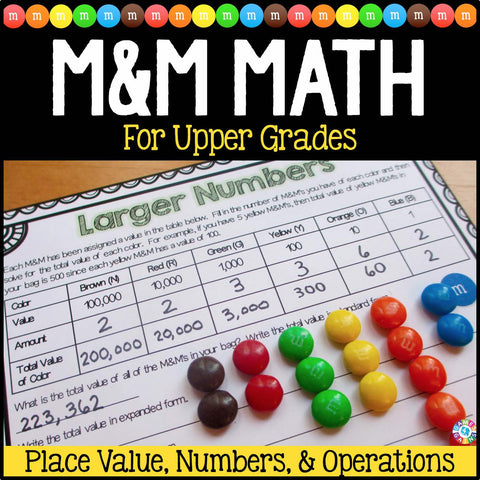## M&M's Math Project

• \$550

Just in time for Halloween, this M&M's Math Project contains 20 pages of printable math activities to use with some yummy M&M's! Grab some bags of M&M's and get ready for your students to have tons of fun practicing number sense, place value, and operations! These work perfectly for small groups, centers, informal assessments, review, etc. Ideal for grades 4-6.

Included with this M&M's Math Project:
Instructions for setup and how to play
20 activity pages

• Numbers Within 1,000 (Place Value)
• Larger Numbers (Place Value)
• Decimal Numbers (Place Value)
• Number Sense – Up to 3 Digits
• Number Sense – Larger Numbers
• Number Sense – Decimals
• One-Step Number Patterns with Addition and Subtraction
• Two-Step Number Patterns with Addition, Subtraction, and Multiplication
• Number Patterns with Decimal Addition and Subtraction
• Addition and Subtraction Within Thousands
• Addition and Subtraction Within Millions
• Addition and Subtraction With Decimals
• Multiplication Arrays
• Multiplication Facts
• Multiplication with Larger Numbers
• Multiplication with Decimals
• Order of Operations
• Factors and Multiples
• Multi-Step Word Problems
• Math with Nutrition Facts

Answer keys are not included since most problems depend on each student's bag of M&M's.

Want to learn more? Be sure to check out the preview for samples of the actual pages and to see the level of the problems included.